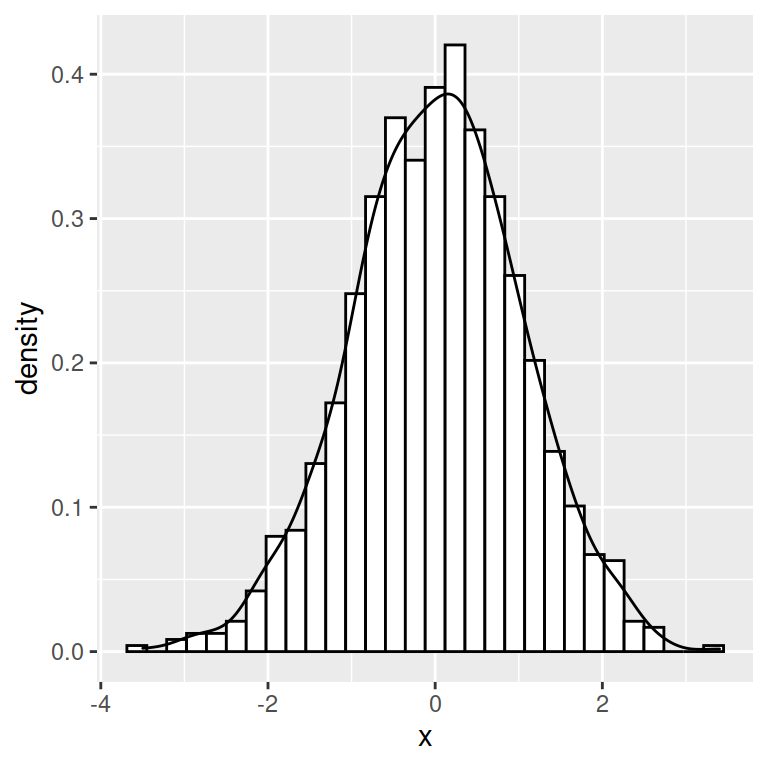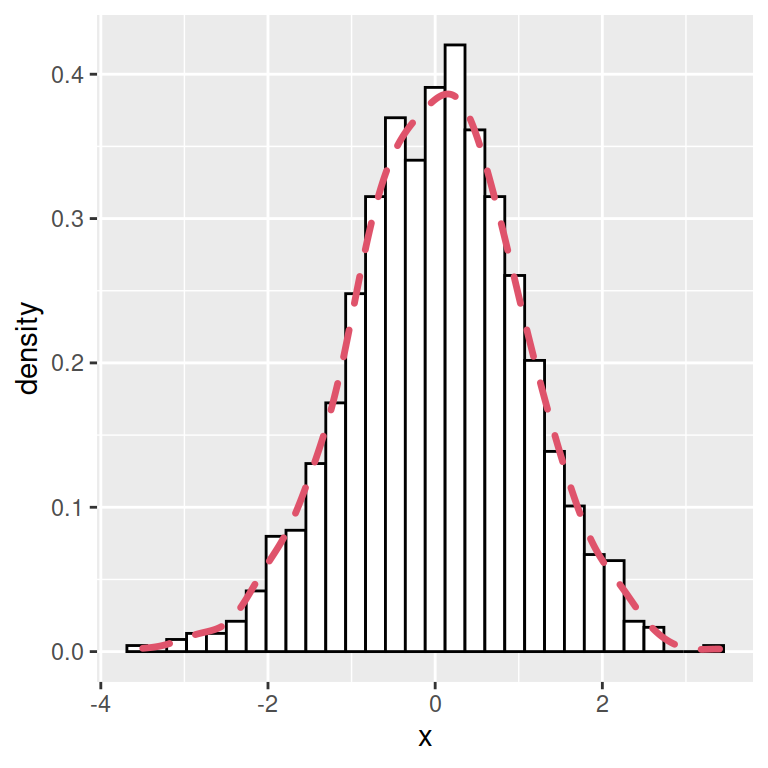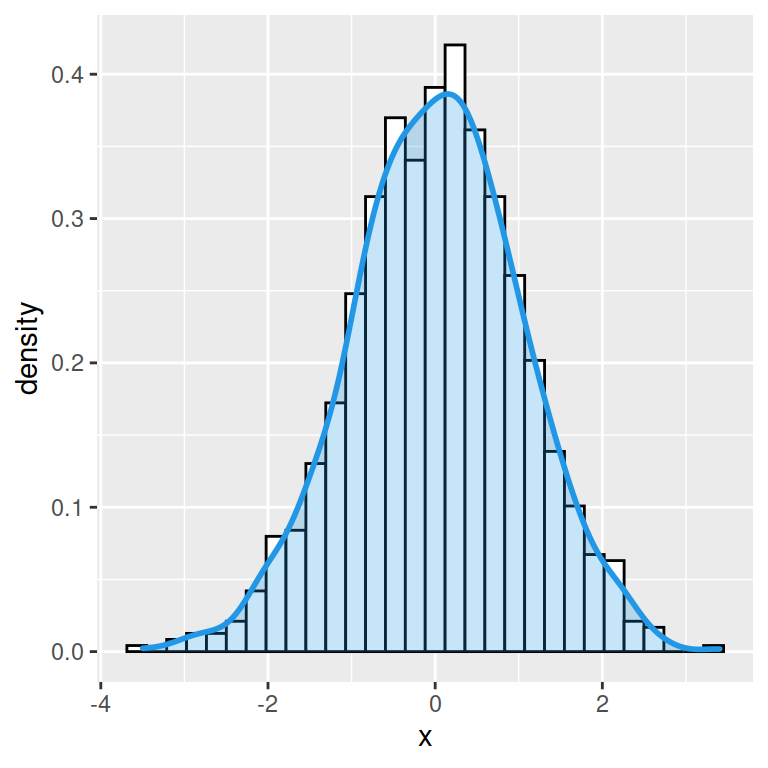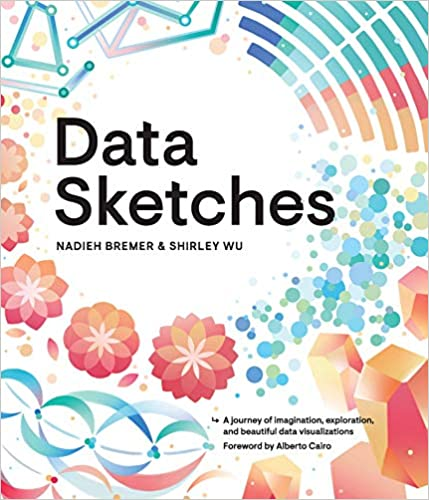# Histogram with density in ggplot2

## Histogram with kernel density estimation

In order to overlay a kernel density estimate over a histogram in ggplot2 you will need to pass `aes(y = ..density..)` to `geom_histogram` and add `geom_density` as in the example below.

``````# install.packages("ggplot2")
library(ggplot2)

# Data
set.seed(5)
x <- rnorm(1000)
df <- data.frame(x)

# Histogram with kernel density
ggplot(df, aes(x = x)) +
geom_histogram(aes(y = ..density..),
colour = 1, fill = "white") +
geom_density()``````## Curve customization

The color, line width and line type of the kernel density curve can be customized making use of `colour`, `lwd` and `linetype` arguments.``````# install.packages("ggplot2")
library(ggplot2)

# Data
set.seed(5)
x <- rnorm(1000)
df <- data.frame(x)

# Histogram with kernel density
ggplot(df, aes(x = x)) +
geom_histogram(aes(y = ..density..),
colour = 1, fill = "white") +
geom_density(lwd = 1.2,
linetype = 2,
colour = 2)``````

## Density curve with shaded area

You can also shade the area behind the curve, specifying a fill color with the `fill` argument of the `geom_density` function. It is recommended to set a level of transparency (between 0 and 1) with `alpha` argument, so the histogram will keep visible.

``````# install.packages("ggplot2")
library(ggplot2)

# Data
set.seed(5)
x <- rnorm(1000)
df <- data.frame(x)

# Histogram with kernel density
ggplot(df, aes(x = x)) +
geom_histogram(aes(y = ..density..),
colour = 1, fill = "white") +
geom_density(lwd = 1, colour = 4,
fill = 4, alpha = 0.25)``````A journey of imagination, exploration, and beautiful data visualizations